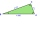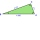# Right triangle calculator - result

Please enter two properties of the right triangle

Use symbols: a, b, c, A, B, h, T, p, r, R

You have entered cathetus b.

### Right scalene triangle.

Sides: a = 6   b = 7.416   c = 9.53992377054

Area: T = 22.248
Perimeter: p = 22.95552377054
Semiperimeter: s = 11.47876188527

Angle ∠ A = α = 38.97549881449° = 38°58'30″ = 0.68802418691 rad
Angle ∠ B = β = 51.02550118551° = 51°1'30″ = 0.89105544577 rad
Angle ∠ C = γ = 90° = 1.57107963268 rad

Height: ha = 7.416
Height: hb = 6
Height: hc = 4.66545236626

Median: ma = 87.9998159979
Median: mb = 7.0533315816
Median: mc = 4.77696188527

Vertex coordinates: A[9.53992377054; 0] B[0; 0] C[3.77438864584; 4.66545236626]
Centroid: CG[4.43877080546; 1.55548412209]
Coordinates of the circumscribed circle: U[4.77696188527; -0]
Coordinates of the inscribed circle: I[4.06216188527; 1.93883811473]

Exterior (or external, outer) angles of the triangle:
∠ A' = α' = 141.0255011855° = 141°1'30″ = 0.68802418691 rad
∠ B' = β' = 128.9754988145° = 128°58'30″ = 0.89105544577 rad
∠ C' = γ' = 90° = 1.57107963268 rad

# How did we calculate this triangle?

The calculation of the triangle progress in two phases. The first phase is such that we try to calculate all three sides of the triangle from the input parameters. The first phase is different for the different triangles query entered. The second phase is the calculation of other characteristics of the triangle, such as angles, area, perimeter, heights, the center of gravity, circle radii, etc. Some input data also results in two to three correct triangle solutions (e.g., if the specified triangle area and two sides - typically resulting in both acute and obtuse) triangle).

### 1. Input data entered: and cathetus b

$a = 0 \ \\ b = 7.416$

### 2. From cathetus b we calculate hypotenuse c - Pythagorean theorem:

$c^2 = a^2+b^2 \ \\ c = \sqrt{ a^2+b^2 } = \sqrt{ 6^2 + 7.416^2 } = \sqrt{ 90.997 } = 9.539$

Now we know the lengths of all three sides of the triangle, and the triangle is uniquely determined. Next, we calculate another its characteristics - same procedure as calculation of the triangle from the known three sides SSS.

$a = 6 \ \\ b = 7.42 \ \\ c = 9.54$

### 3. The triangle perimeter is the sum of the lengths of its three sides

$p = a+b+c = 6+7.42+9.54 = 22.96$

### 4. Semiperimeter of the triangle

The semiperimeter of the triangle is half its perimeter. The semiperimeter frequently appears in formulas for triangles that it is given a separate name. By the triangle inequality, the longest side length of a triangle is less than the semiperimeter.

$s = \dfrac{ p }{ 2 } = \dfrac{ 22.96 }{ 2 } = 11.48$

### 5. The triangle area - from two legs

$T = \dfrac{ ab }{ 2 } = \dfrac{ 6 \cdot \ 7.42 }{ 2 } = 22.25$

### 6. Calculate the heights of the right triangle from its area.

$h _a = b = 7.42 \ \\ \ \\ h _b = a = 6 \ \\ \ \\ T = \dfrac{ c h _c }{ 2 } \ \\ \ \\ \ \\ h _c = \dfrac{ 2 \ T }{ c } = \dfrac{ 2 \cdot \ 22.25 }{ 9.54 } = 4.66$

### 7. Calculation of the inner angles of the triangle - basic use of sine function

$\sin α = \dfrac{ a }{ c } \ \\ α = \arcsin(\dfrac{ a }{ c } ) = \arcsin(\dfrac{ 6 }{ 9.54 } ) = 38^\circ 58'30" \ \\ \sin β = \dfrac{ b }{ c } \ \\ β = \arcsin(\dfrac{ b }{ c } ) = \arcsin(\dfrac{ 7.42 }{ 9.54 } ) = 51^\circ 1'30" \ \\ γ = 90^\circ$

An incircle of a triangle is a circle which is tangent to each side. An incircle center is called incenter and has a radius named inradius. All triangles have an incenter, and it always lies inside the triangle. The incenter is the intersection of the three angle bisectors. The product of the inradius and semiperimeter (half the perimeter) of a triangle is its area.

$T = rs \ \\ r = \dfrac{ T }{ s } = \dfrac{ 22.25 }{ 11.48 } = 1.94$

The circumcircle of a triangle is a circle that passes through all of the triangle's vertices, and the circumradius of a triangle is the radius of the triangle's circumcircle. Circumcenter (center of circumcircle) is the point where the perpendicular bisectors of a triangle intersect.

$R = \dfrac{ c }{ 2 } = \dfrac{ 9.54 }{ 2 } = 4.77$

### 10. Calculation of medians

A median of a triangle is a line segment joining a vertex to the midpoint of the opposite side. Every triangle has three medians, and they all intersect each other at the triangle's centroid. The centroid divides each median into parts in the ratio 2:1, with the centroid being twice as close to the midpoint of a side as it is to the opposite vertex. We use Apollonius's theorem to calculate the length of a median from the lengths of its side.

$m_a^2 = b^2 + (a/2)^2 \ \\ m_a = \sqrt{ b^2 + (a/2)^2 } = \sqrt{ 7.42^2 + (6/2)^2 } = 8 \ \\ \ \\ m_b^2 = a^2 + (b/2)^2 \ \\ m_b = \sqrt{ a^2 + (b/2)^2 } = \sqrt{ 6^2 + (7.42/2)^2 } = 7.053 \ \\ \ \\ m_c = R = \dfrac{ c }{ 2 } = \dfrac{ 9.54 }{ 2 } = 4.77$

The right triangle calculators compute angles, sides (adjacent, opposite, hypotenuse) and area of any right-angled triangle and use it in the real world. Two independent properties entirely determine any right-angled triangle. The calculator provides a step-by-step explanation for each calculation.

A right triangle is a kind of triangle that has one angle that measures C=90°. In a Right triangle, the side c that is opposite of the C=90° angle, is the longest side of the triangle and is called the hypotenuse. The variables a, b are the lengths of the shorter sides, also called legs or arms. Variables for angles are A, B, or α (alpha) and β (beta). Variable h refers to the altitude(height) of the triangle, which is the length from the vertex C to the hypotenuse of the triangle.

Examples for right triangle calculation:

## A right triangle in word problems in mathematics:

• Triangle P2Can triangle have two right angles?
• Height 2Calculate the height of the equilateral triangle with side 38.
• The ditchDitch with cross section of an isosceles trapezoid with bases 2m 6m are deep 1.5m. How long is the slope of the ditch?
• Vector 7Given vector OA(12,16) and vector OB(4,1). Find vector AB and vector |A|.
• Triangle ABCIn a triangle ABC with the side BC of length 2 cm The middle point of AB. Points L and M split AC side into three equal lines. KLM is isosceles triangle with a right angle at the point K. Determine the lengths of the sides AB, AC triangle ABC.
• RT triangle and heightCalculate the remaining sides of the right triangle if we know side b = 4 cm long and height to side c h = 2.4 cm.
• Area of RT 2Calculate the area of right triangle whose legs have a length 5.8 cm and 5.8 cm.
• Four ropesTV transmitter is anchored at a height of 44 meters by four ropes. Each rope is attached at a distance of 55 meters from the heel of the TV transmitter. Calculate how many meters of rope were used in the construction of the transmitter. At each attachment
• Spruce heightHow tall was spruce that was cut at an altitude of 8m above the ground and the top landed at a distance of 15m from the heel of the tree?
• CablewayCableway has a length of 1800 m. The horizontal distance between the upper and lower cable car station is 1600 m. Calculate how much meters altitude is higher upper station than the base station.
• ChaunceyChauncey is building a storage bench for his son’s playroom. The storage bench will fit into the corner and against two walls to form a triangle. Chanuncy wants to buy a triangular shaped cover for the bench. If the storage bench is 2 1/2 ft. Along one
• BisectorsAs shown, in △ ABC, ∠C = 90°, AD bisects ∠BAC, DE⊥AB to E, BE = 2, BC = 6. Find the perimeter of triangle △ BDE.
• Right triangleRight triangle legs has lengths 630 mm and 411 dm. Calculate the area of this triangle.
• CalculateCalculate the length of a side of the equilateral triangle with an area of 50cm2.
• Broken treeThe tree is broken at 4 meters above the ground and the top of the tree touches the ground at a distance of 5 from the trunk. Calculate the original height of the tree.